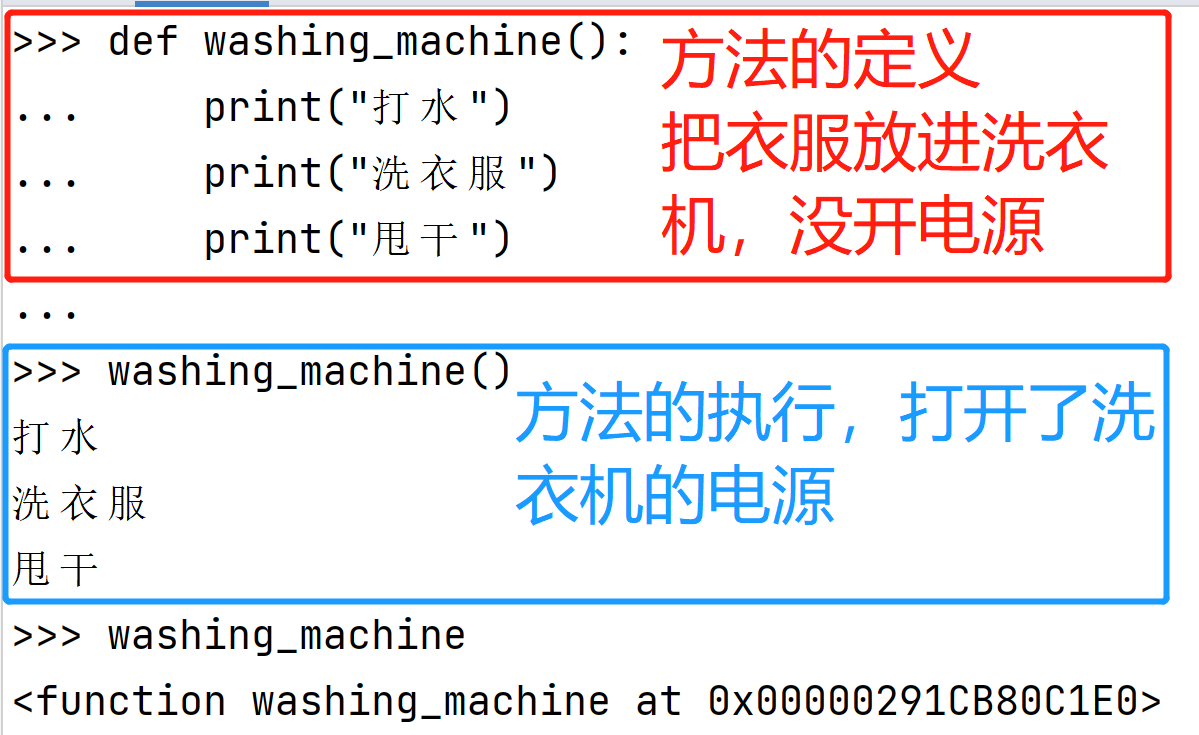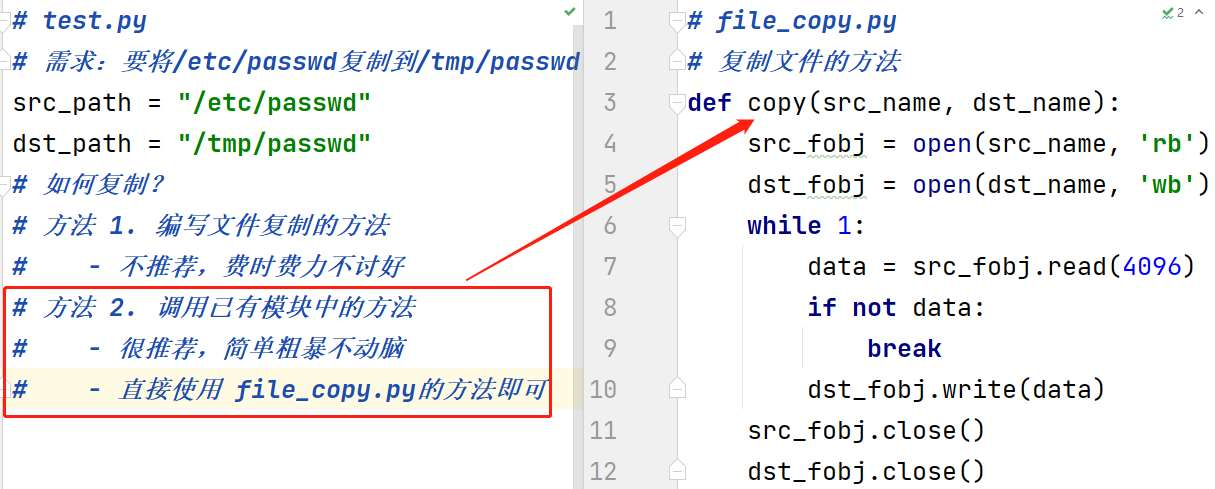﻿ Python中函数的创建与调用你了解吗_python_脚本之家
python# Python中函数的创建与调用你了解吗

## 创建函数

```def 函数名(参数列表):  # 具体情况具体对待，参数可有可无
"""函数说明文档字符串"""
函数封装的代码
……```

`def` 子句的剩余部分包括了一个虽然可选但是强烈推荐的文档字串，和必需的函数体

• 可以由 字母、下划线 和 数字 组成
• 不能以数字开头
• 不能与关键字重名
```def washing_machine():  # 洗衣机可以帮我们完成
print("打水")
print("洗衣服")
print("甩干")
```

### 调用函数```def washing_machine():  # 洗衣机可以帮我们完成
print("打水")
print("加洗衣粉！！！")
print("洗衣服")
print("甩干")
# 早上洗衣服
washing_machine()
# 中午洗衣服
washing_machine()
# 晚上洗衣服
washing_machine()```• 定义好函数之后，只表示这个函数封装了一段代码而已
• 如果不主动调用函数，函数是不会主动执行的

• 能否将 函数调用 放在 函数定义 的上方？
• 不能！
• 因为在 使用函数名 调用函数之前，必须要保证 `Python` 已经知道函数的存在
• 否则控制台会提示 `NameError: name 'menu' is not defined` (名称错误：menu 这个名字没有被定义)

## 函数的参数

### 形参和实参

• 形参定义 函数时，小括号中的参数，是用来接收参数用的，在函数内部 作为变量使用
• 实参调用 函数时，小括号中的参数，是用来把数据传递到 函数内部 用的

```def washing_machine():  # 洗衣机可以帮我们完成
print("打水")
print("加洗衣粉！！！")
print("洗床单")  # 洗被套
print("甩干")```

```def washing_machine():  # 洗衣机可以帮我们完成
print("打水")
print("加洗衣粉！！！")
print("洗衣服")
print("甩干")
washing_machine()
def washing_machine():  # 洗衣机可以帮我们完成
print("打水")
print("加洗衣粉！！！")
print("洗床单")
print("甩干")
washing_machine()
......```

### 传递参数

• 在函数名的后面的小括号内部填写 参数
• 多个参数之间使用 `,` 分隔
• 调用函数时，实参的个数需要与形参个数一致，实参将依次传递给形参
```def washing_machine(something):  # 洗衣机可以帮我们完成
print("打水")
print("加洗衣粉！！！")
print("洗" + something)
print("甩干")
# 洗衣服
washing_machine("衣服")
# 洗床单
washing_machine("床单")``````def washing_machine(xidiji,something):  # 洗衣机可以帮我们完成
print("打水")
print("加" + xidiji)
print("洗衣服" + something)
print("甩干")
#早上洗衣服
#按照参数位置顺序传递参数的方式叫做位置传参
#使用洗衣机，执行洗衣机内部的逻辑
washing_machine("洗衣液","衣服")#something = 衣服
#中午洗被罩
washing_machine("洗衣粉","被罩")# something = 被罩
#晚上洗床单
washing_machine("五粮液","床单")# something = 床单```

• 函数，把 具有独立功能的代码块 组织为一个小模块，在需要的时候 调用
• 函数的参数，增加函数的 通用性，针对 相同的数据处理逻辑，能够 适应更多的数据

1.在函数 内部，把参数当做 变量 使用，进行需要的数据处理

2.函数调用时，按照函数定义的参数顺序，把 希望在函数内部处理的数据，通过参数 传递

### 位置参数## 函数的返回值

• 在程序开发中，有时候，会希望 一个函数执行结束后，告诉调用者一个结果，以便调用者针对具体的结果做后续的处理
• 返回值 是函数 完成工作后，最后 给调用者的 一个结果
• 在函数中使用 `return` 关键字可以返回结果
• 调用函数一方，可以 使用变量 来 接收 函数的返回结果

### 案例：计算任意两个数字的和

```# 函数的返回值: return, 用于对后续逻辑的处理
# 理解: 把结果揣兜里，后续想干啥干啥，想打印打印，想求和就求和
# 注意:
# a. 函数中如果没有return，那么解释器会自动加一个return None
# b. return表示函数的终止，return后的代码都不会执行
# 1 定义一个函数，计算两个数的和
# 2 计算这两个数的和是不是偶数
def get_sum(x, y=100):  # 默认参数
he = x + y  # sum = 10 + 20
return he   # return 30
print("return表示函数的终止，return后的代码都不会执行")
# 将函数return后的结果赋值给变量dc: dc = sum  ->  dc = 30
dc = get_sum(10, 20)  # x = 10, y = 20
print("dc:", dc)  # 30
dc1 = get_sum(10)  # x = 10, y = 100
print("dc1:", dc1)  # 110
# if dc % 2 == 0:
#     print("偶数")
# else:
#     print("奇数")
```
```#默认参数
#注意：具有默认值的参数后面不能跟没有默认值的参数
def get_sum(a=20,b=5,c=10):
he = a + b+ c
return he

dc = get_sum(1,2,3) #a=1 b=2 c=3
print("dc:",dc) # 6
dc1 = get_sum(1,2)  # a=1 b=2 c=10
print("dc1:",dc1) # 13
dc2 = get_sum(1)  # a=1 b=5 c=10
print("dc2:",dc2) # 16
dc3 = get_sum()
print("dc3:",dc3) # 35```修改菲波那切数列

```def new_fib(n=8):
list01 = [0,1]  #定义列表，指定初始值
for dc in range(n-2):
list01.append(list01[-1]+list01[-2])
return list01

dc = new_fib()   #不加参数默认是8
dc1 = new_fib(10)
print("dc:",dc)
print("dc1:",dc1)``````#练习：生成随机密码
#创建 randpass.py 脚本，要求如下：
#编写一个能生成8位随机密码的程序
#使用 random 的 choice 函数随机取出字符
#由用户决定密码长度
import random
n = int(input("密码长度："))
all = "0123456789zxcvbnmlkjhgfdsaqwertyuiopPOIUYTREWQASDFGHJKLMNBVCXZ" # 0-9 a-z A-Z
random.choice(all)
for i in range(n):
dc = random.choice(all)
# 调用函数，才能执行函数内部逻辑
print("dc:",dc)``````#练习：生成随机密码
#创建 randpass.py 脚本，要求如下：
#编写一个能生成8位随机密码的程序
#使用 random 的 choice 函数随机取出字符
#由用户决定密码长度
import random,string
n = int(input("密码长度："))
all = string.ascii_letters + string.digits
random.choice(all)
for i in range(n):
dc = random.choice(all)
# 调用函数，才能执行函数内部逻辑
print("dc:",dc)```## 模块基础

### 定义模块

#### 基本概念

• 模块是从逻辑上组织python代码的形式
• 当代码量变得相当大的时候，最好把代码分成一些有组织的代码段，前提是保证它们的 彼此交互
• 这些代码片段相互间有一定的联系，可能是一个包含数据成员和方法的类，也可能是一组相关但彼此独立的操作函数

### 导入模块 (import)

• 使用 import 导入模块
• 模块属性通过 “模块名.属性” 的方法调用
• 如果仅需要模块中的某些属性，也可以单独导入```# test.py，将 file_copy.py 放在同级目录下
# 需求：要将/etc/passwd复制到/tmp/passwd
src_path = "/etc/passwd"
dst_path = "/tmp/passwd"
# 如何复制？
# 调用已有模块中的方法
#    - 很推荐，简单粗暴不动脑
#    - 直接使用 file_copy.py的方法即可

# 导入方法一：直接导入模块
import file_copy  # 要注意路径问题
file_copy.copy(src_path, dst_path)

# 导入方法二：只导入 file_copy 模块的 copy 方法
from file_copy import copy  # 如果相同时导入多个模块 from file_copy import *
copy(src_path, dst_path)

# 导入方法四：导入模块起别名 as
import file_copy as fc
fc.copy(src_path, dst_path)```

• 一行指导入一个模块，可以导入多行， 例如：`import random`
• 只导入模块中的某些方法，例如：`from random import choice`, `randint`

• 一个模块只被 加载一次，无论它被导入多少次
• 只加载一次可以 阻止多重导入时，代码被多次执行
• 如果两个文件相互导入，防止了无限的相互加载
• 模块加载时，顶层代码会自动执行，所以只将函数放入模块的顶层是最好的编程习惯

### 模块特性及案例

#### 模块特性

```# foo.py
print(__name__)

# bar.py
import foo  # 导入foo.py，会将 foo.py 中的代码完成的执行一次，所以会执行 foo 中的 print(__name__)```

# foo.py   ->   __main__   当模块文件直接执行时，__name__的值为‘__main__’
# bar.py   ->   foo        当模块被另一个文件导入时，__name__的值就是该模块的名字```def test():
......
if __name__ == "__main__":
test()```